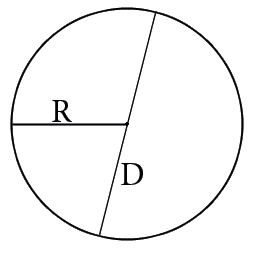# Area of a circle calculator.

You could easy calculate circle's area by our online calculator.Calculate the area of a circle by: R - radiusD - diameter Length of the radius R:

You need to know circle's radius or diameter for you will be able to calculate it's area. If you have one of mentioned values it won't be a problem to calculate the area.
Сircle's area is calculated by the next formulas:

1. If you know the radius:
S=πR2
2. If you know the diameter:
S=πD2/4

where S – area, R – radius, D – diameter, π – pi constant which equal about 3,14.

### Circle's areaHOME
RESOURCES